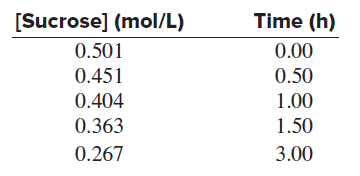# Problem: The acid-catalyzed hydrolysis of sucrose occurs by the following overall reaction, whose kinetic data are given below:C12H22O11(s) + H2O(l) ⟶C6H12O6(aq) + C6H12O6(aq)     sucrose                         glucose           fructose(a) Determine the rate constant and the half-life of the reaction.

⚠️Our tutors found the solution shown to be helpful for the problem you're searching for. We don't have the exact solution yet.

###### Problem Details

The acid-catalyzed hydrolysis of sucrose occurs by the following overall reaction, whose kinetic data are given below:

C12H22O11(s) + H2O(l) ⟶C6H12O6(aq) + C6H12O6(aq)
sucrose                         glucose           fructose(a) Determine the rate constant and the half-life of the reaction.